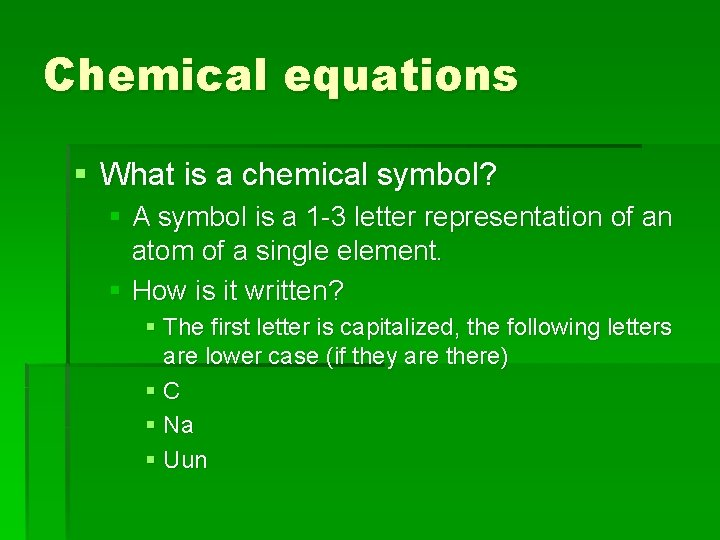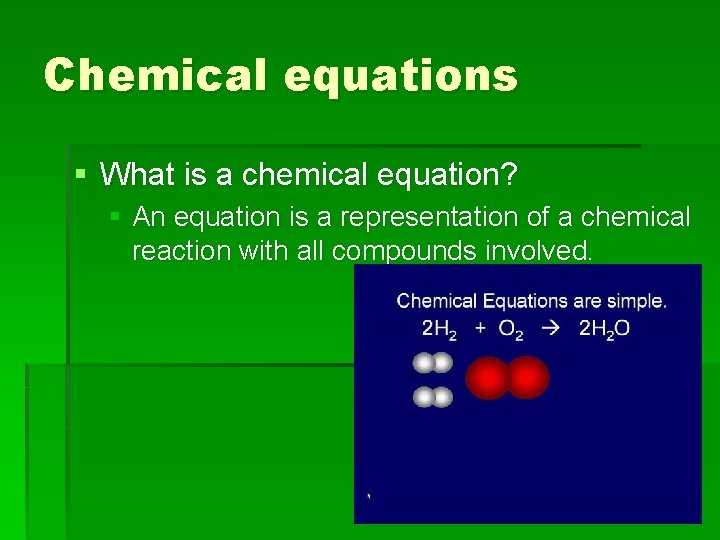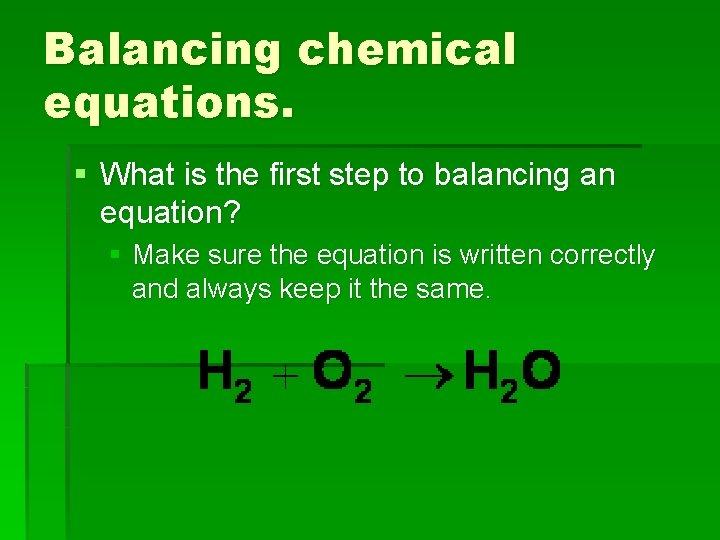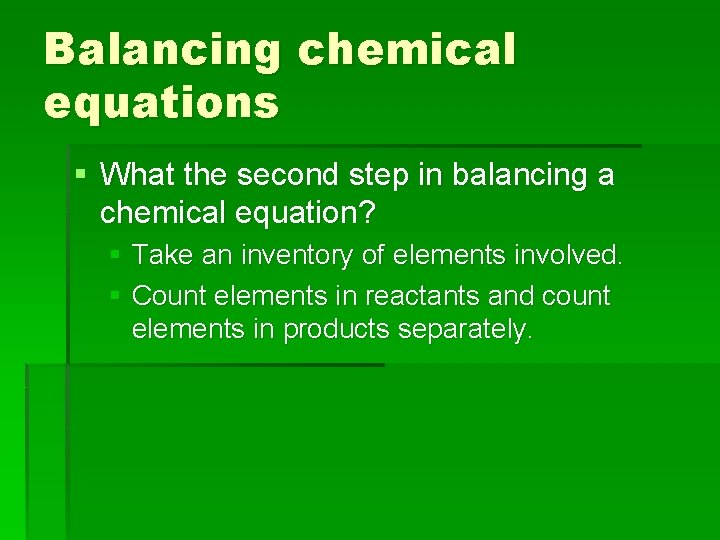Chemical equations Reactions Chemical equations What is a

• Slides: 9Chemical equations Reactions!!!!Chemical equations § What is a chemical symbol? § A symbol is a 1 -3 letter representation of an atom of a single element. § How is it written? § The first letter is capitalized, the following letters are lower case (if they are there) §C § Na § UunChemical equations § What is a chemical formula? § A formula is a representation of a compound (bonds) using chemical symbols. § How is a formula written? § Each symbol represents one atom, and each subscript multiplies the atoms by that number. § Na. Cl § H 2 OChemical equations § What is a chemical equation? § An equation is a representation of a chemical reaction with all compounds involved.Chemical equations § What are the parts to a chemical equation? § How is this equation read?Chemical equations § What is the law of conservation of mass? § This law states that under ordinary circumstances, matter can be neither created nor destroyed. § What does this mean for chemical equations?Balancing chemical equations. § What is the first step to balancing an equation? § Make sure the equation is written correctly and always keep it the same.Balancing chemical equations § What the second step in balancing a chemical equation? § Take an inventory of elements involved. § Count elements in reactants and count elements in products separately.Balancing chemical equations § What is the final step in balancing a chemical equation? § Use coefficients to make the number of elements on each side equal. § You cannot change a chemical formula.